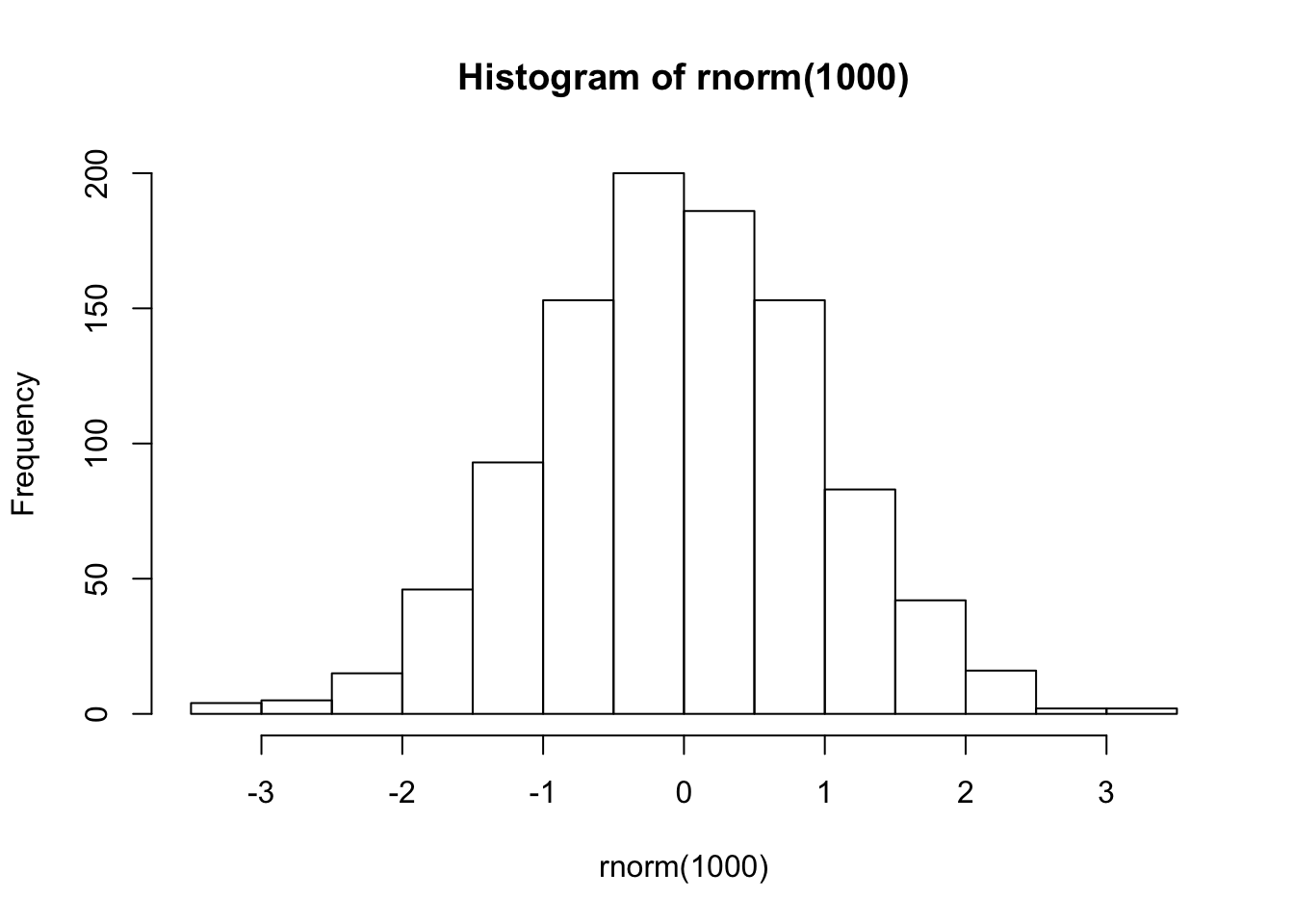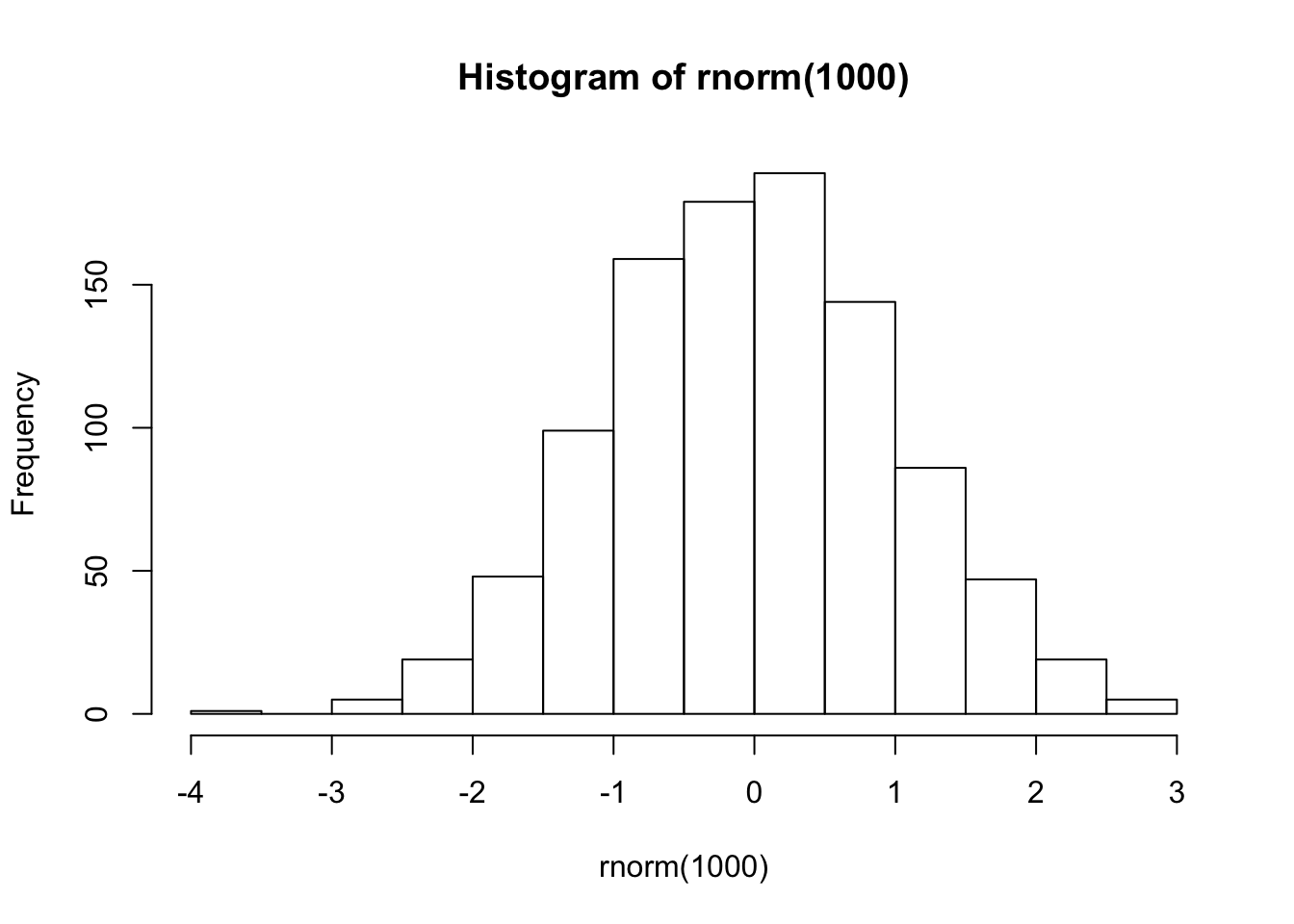# 1 Goal

The goal of this tutorial is to understand the use of the SetSeed function and key concepts like random number generation with R.

# 2 What is the function SetSeed

``````# A computer is not able to create true random numbers, but pseudo random numbers
# To optimize this process inside the computer there is actually a list of random numbers
# The random component is where in this list we start to generate numbers
# Forcing the seed to be the same will generate exactly the same list of random numbers
# Remember that the position of the seed is defined by you
# Then we will be able to compare performances removing the random effects

# We start the list of random numbers at the position 123
set.seed(123)``````

# 3 Sample from a vector

``````# Sampling a vector is a random procedure
# We want 5 random numbers from 1 to 10
sample(1:10, 5)``````
``##  3 8 4 7 6``
``````# Each time we sample we get a different list of numbers
sample(1:10, 5)``````
``##  1 5 8 4 3``
``sample(1:10, 5)``
``##  10  5  6  9  1``
``sample(1:10, 5)``
``##   9  3  1 10  6``
``````# However we can obtain always the same sample by forcing the seed
set.seed(123)
# Notice that it's the same sample we got on the first attempt
sample(1:10, 5)``````
``##  3 8 4 7 6``
``````# And we get the same sample over and over again
set.seed(123)
sample(1:10, 5)``````
``##  3 8 4 7 6``
``````set.seed(123)
sample(1:10, 5)``````
``##  3 8 4 7 6``
``````set.seed(123)
sample(1:10, 5)``````
``##  3 8 4 7 6``

# 4 Normal distribution

``````# We can generate a normal distribution with random entries
hist(rnorm(1000))````````````# Each time that we generate the distribution is slightly different
hist(rnorm(1000))````````hist(rnorm(1000))``